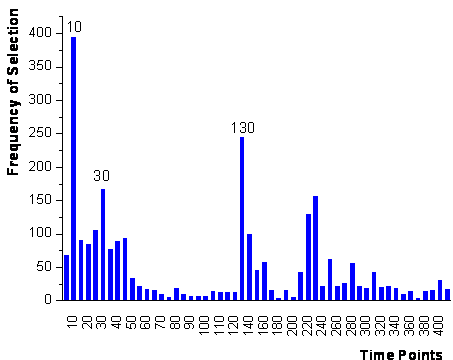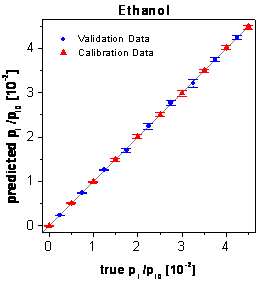Home News About Me Ph. D. Thesis Abstract Table of Contents 1. Introduction 2. Theory  Fundamentals of the Multivariate Data Analysis 3. Theory  Quantification of the Refrigerants R22 and R134a: Part I 4. Experiments, Setups and Data Sets 5. Results  Kinetic Measurements 6. Results  Multivariate Calibrations 7. Results  Genetic Algorithm Framework 8. Results  Growing Neural Network Framework 9. Results  All Data Sets 9.1. Methanol and Ethanol by SPR 9.1.1. Single Analytes 9.1.2. Parallel Growing Neural Network Framework 9.1.3. Sensitivity Analysis 9.1.4. Brute Force Variable Selection 9.1.5. Conclusions 9.2. Methanol, Ethanol and 1-Propanol by SPR 9.3. Methanol, Ethanol and 1-Propanol by the RIfS Array and the 4l Setup 9.4. Quaternary Mixtures by the SPR Setup and the RIfS Array 9.5. Quantification of the Refrigerants R22 and R134a in Mixtures: Part II 10. Results  Various Aspects of the Frameworks and Measurements 11. Summary and Outlook 12. References 13. Acknowledgements Publications Research Tutorials Downloads and Links Contact Search Site Map Print this Page### 9.1.2.   Parallel Growing Neural Network Framework

For the multivariate calibration, neural networks using all time points of the calibration data were trained set and subsequently predicted the validation data set. The root mean square errors of the crossvalidation and of the prediction of the independent validation data set are listed in the first row of table 5. The concentrations of the test samples were predicted with a relative RMSE of 3.32% for methanol and of 4.11% for ethanol. The fully connected networks used for the calibration consisted of 53 input neurons, 4 hidden neurons and 1 output neuron. In order to improve the calibration, the parallel growing neural network framework introduced in chapter 8 was applied to the calibration data.  Thereby the growing network algorithm was repeated 200 times for each analyte. In contrast to chapter 8, the frequencies of the variables being selected after the first step of the algorithm are combined for both analytes by summing up the individual frequencies of each analyte. The corresponding plot (figure 60) shows that the variables in three time intervals are prominent: The beginning of exposure to analyte (10-45 s), when exposure to analyte has ended and exposure to synthetic air starts again (130-140 s), and additionally hundred seconds after the start of exposure to synthetic air (220-230 s). The first two intervals are easily interpretable as in accordance with figure 15 the sensor responses for both analytes differ most during these time intervals. The time interval around 220 s might be considered as reference signal with practically no analyte remaining to compensate possible drifts of the baseline.

 Number of Time Points Calibration Data Validation Data Methanol Ethanol Methanol Ethanol 53 4.77 3.14 3.32 4.11 3 2.01 1.91 1.97 2.37 2 2.28 2.36 2.22 2.70

table 5:      Relative RMSE in % for the prediction by the neural networks using different time points.figure 60:  Ranking of the time points after the first step of the parallel framework for both analytes together.

The final model obtained by a stepwise addition of time points (step 2 in figure 53) uses only the 3 time points 10 s, 30 s and 130 s (topology 3-4-1). According to table 5 the prediction errors are significantly lower compared with the fully connected networks for both, the crossvalidated calibration data and for the validation data with excellent low errors of 1.97% for methanol and 2.37 % for ethanol. The third time interval is not used by this network and consequently seems not to contain significant additional information not covered by the 3 other sensor signals. Using reproduced measurements of the single analytes in the same concentration range, a standard deviation of the signals was calculated with 0.62% for methanol and 0.98 % for ethanol. These errors are caused by the noise of the spectrometer, inaccuracies of the gas mixing station and fluctuations of the temperature and thus also exist for the measurements of the mixtures. The only moderate increase of the errors for the calibration and prediction shows the potential of the calibration and variable selection by the growing neural network framework. In figure 61 the true-predicted plots of the validation data and the calibration data are shown. The predictions of all concentrations are characterized by very small standard deviations and by the absence of systematic errors.figure 61:  Predictions of the calibration and validation data by the optimized neural networks using only three time points.

 Page 116 © Frank Dieterle, 03.03.2019